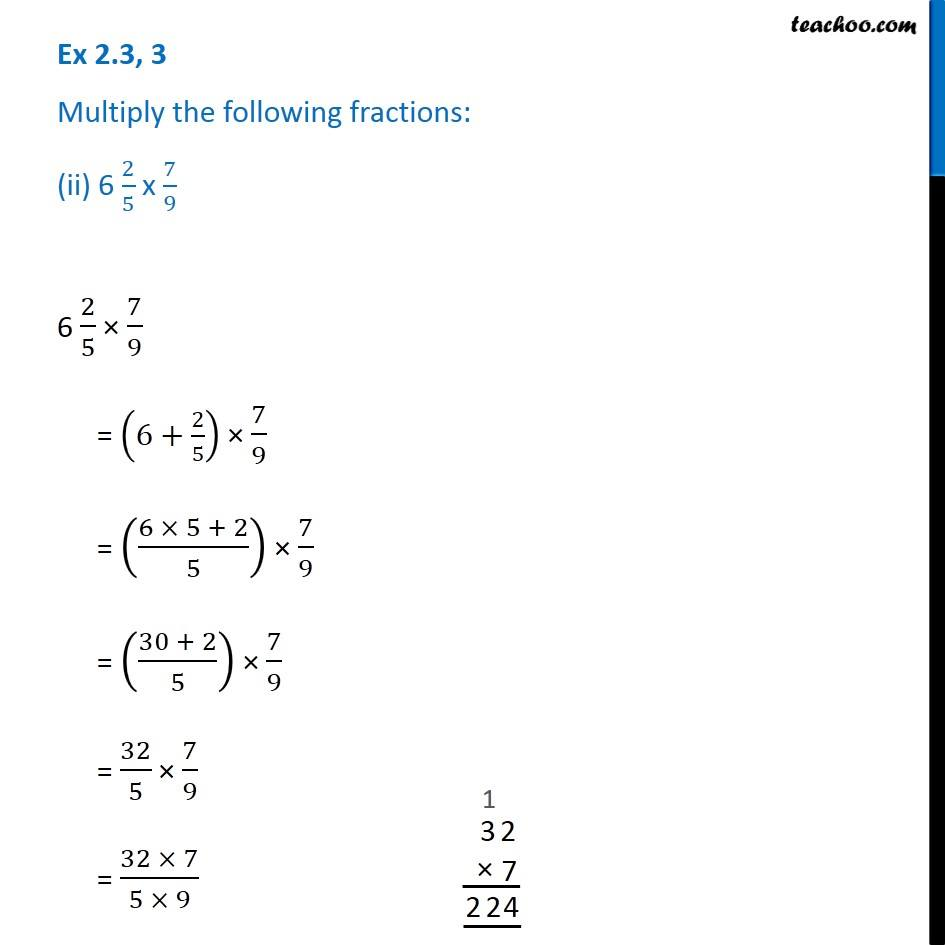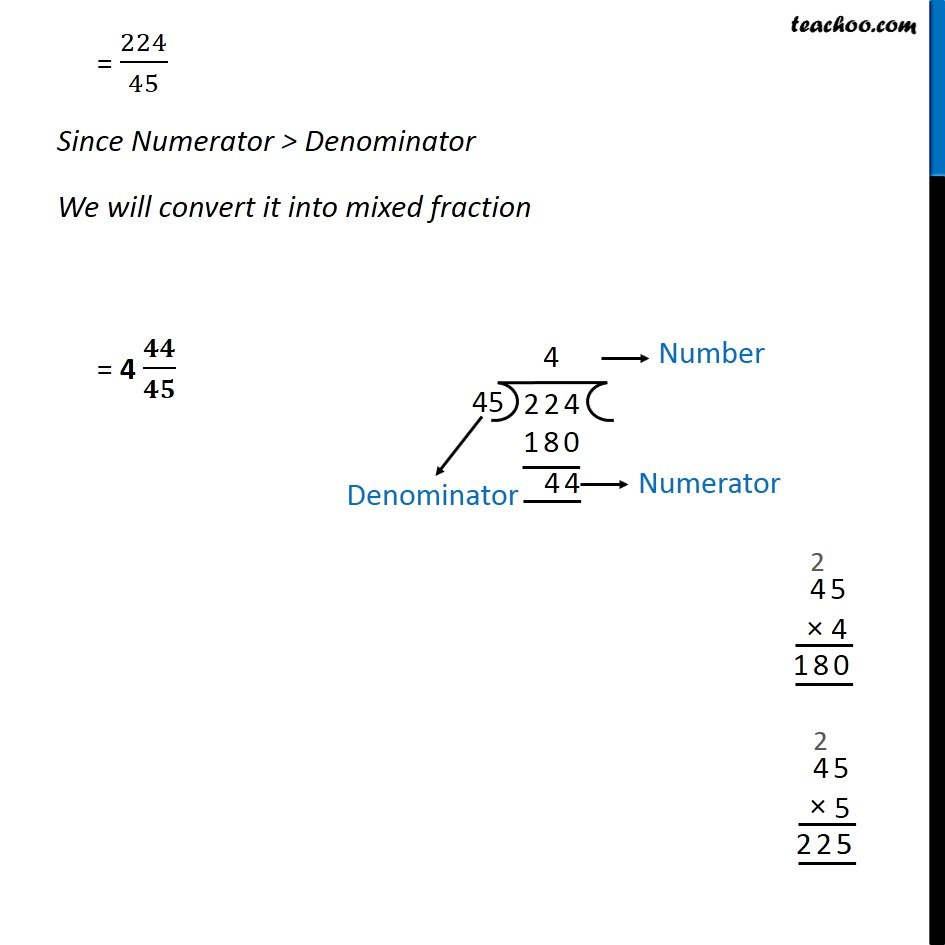Ex 2.3

Chapter 2 Class 7 Fractions and Decimals
Serial order wiseGet live Maths 1-on-1 Classs - Class 6 to 12

### Transcript

Ex 2.3, 3 Multiply the following fractions: (ii) 6 2/5 x 7/9 6 2/5 × 7/9 = (6+2/5) × 7/9 = ((6 × 5 + 2)/5) × 7/9 = ((30 + 2)/5) × 7/9 = 32/5 × 7/9 = (32 × 7)/(5 × 9) = 224/45 Since Numerator > Denominator We will convert it into mixed fraction = 4 𝟒𝟒/𝟒𝟓